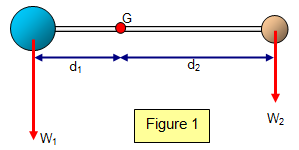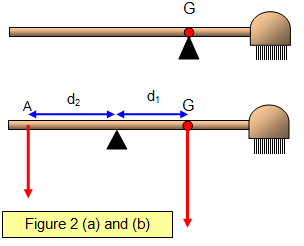# Centre of gravity

The weight of an object may be taken as acting at one point known as the centre of gravity. You could think of that point as the position where all the mass of the object could be thought to be concentrated.

The resultant moment about the centre of gravity of any object must be zero.

The dumbbell arrangement in Figure 1 shows this clearly.(W1 = m1g , W2 = m2g)

Anticlockwise moment = W1 x d1

Clockwise moments = W2 x d2.

Now clearly if all the mass was replaced by a mass of M (= m1+m2) at G there would be no turning effect about G and therefore the resultant moment must be zero.

### Finding the mass of a broom using its centre of gravityIf a broom is balanced on a fulcrum (Figure 2(a)) its centre of gravity can be found. If it is then balanced on a different point (Figure 2.b) with a known force F using the principle of moments:

W x d1 = F x d2

Therefore the weight of the broom is:

Weight of broom (W) = mg = Fx[d1/d2]

And its mass (m) is:

Mass of broom (m) = Fx[d1/gd2]

Example problem
A broom is balanced on a point as shown in Figure 2 and is kept horizontal by a force of 30 N hanging from A, a distance (d2) from the pivot of 0.35 m.
If the centre of gravity of the broom (G) is a distance (d1) from the pivot of 0.55 m calculate the weight of the broom.

Weight = Fx[d2/d1] = 30x[0.35/0.55] = 19 N

### Centre of mass and centre of gravity.

If the gravitational field is uniform over the size of the object then the centre of mass and the centre of gravity of the object will coincide. However if this is not true then they will be in different places. Close to a black hole where the gravitational field changes rapidly might give you a situation like this! This would also mean that a unit mass at one point in an object would have a different force on it from another unit mass somewhere else in the object and so the object would be distorted.

### Replacing a couple by a force through the centre of gravity (G) and a couple about GIn Figure 3 a force F acts at P. This will do two things:

(a) cause the box to move along the line PX and
(b) rotate about the centre of gravity (G)

The moment about G is Fd and so we could replace the force by an equal one acting through G in the x direction and a couple of moment Fd about G. This couple could be provided by any size force acting at any distance from G as long as the product of force and distance was Fd.

A VERSION IN WORD IS AVAILABLE ON THE SCHOOLPHYSICS USB